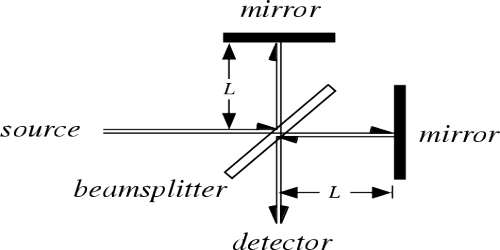# Michelson-Morley Experiment

Michelson-Morley Experiment

After the discovery of Maxwell’s equations in 1861 it was seen that electromagnetic wave propagates in vacuum at the speed of light. Later on, Hertz proved that light is an electromagnetic wave. At that time it was beyond imagination that wave could travel without a material medium. So, it was thought that everywhere in the universe, even in space there is a medium inside atoms and molecules and through which planets, stars move – a medium which does not give resistance to anybody’s motion, does not have weight. This medium was named as ether. With respect to ether stationary frame was called a privileged frame of reference. From Bradley’s experiment, it is known that the earth travels at 30 km/sec with respect to ether medium and does not affect or disturb the surrounding ether medium.

In order to measure the relative velocity between the earth and ether scientists conducted many experiments. But Michelson-Morley’s experiment was a negative type of experiment. So, this experiment created sufficient interest among scientists. In this negative type of experiment, the myth about the ether medium was exposed. Michelson developed an unprecedented sensitive instrument for which he was honored with Nobel Prize. So, this instrument was named as Michelson’s interferometer [Figure]. This is a type of experiment in physics from which it is clearly understood that there is no existence of ether medium.

Michelson and Morley built a Michelson interferometer, which essentially consists of a light source, a half-silvered glass plate, two mirrors, and a telescope. The mirrors are placed at right angles to each other and at equal distance from the glass plate, which is obliquely oriented at an angle of 45° relative to the two mirrors. In the original device, the mirrors were mounted on a rigid base that rotates freely on a basin filled with liquid mercury in order to reduce friction.

In this instrument, S is a monochromatic light from where emitted light after becoming parallel by the lens is incident on a half-silvered glass plate Pi inclined at an angle of 45°. This incident ray is divided into two parts at point A at a right angle. One part after reflecting from the upper surface of P1 perpendicularly falls on the mirror M1 and getting reflected again returns to the telescope T following the same path. The other ray being refracted through the plate P1 is incident perpendicularly on the mirror M2 and after reflecting back meets the first ray. These two light rays travel almost equal path. The fronts surfaces of the mirrors M1 and M2 are silvered perfectly so that multiple reflections do not take place and the two mirrors are arranged perpendicular to each other.Fig: Michelson-Morley Experiment

The distance between both the mirrors and plate P1 is taken as d. Here P2 is a compensating plate by which distance traveled in the glass is made equal for both the rays. If the two light rays become perfectly parallel and distances between plate P1 and AB and AC become equal to d, then reflected rays from M1 and M2 remain in the same

phase and shows bright fringe in the telescope, T. If the angle between M1 and M2 is a right-angle, then interferences pattern will be aggregate of concentric circles, but if the angle between M1 and M2 is less than a right angle, which was kept in the experiment, then the interference pattern will be an aggregate of parallel lines. Suppose the velocity of the instrument with respect to ether medium is – v and in the reverse is – v, if the velocity of light is exactly equal to c, then the velocity of light with respect to the instrument will be (c – v) along AC and if time taken to travel from A to C is t1 then t1T1 = d / (c – v)

The light ray during return after reflecting from the mirror M2 will be (c + v) and time, t2 = d / (c + v).